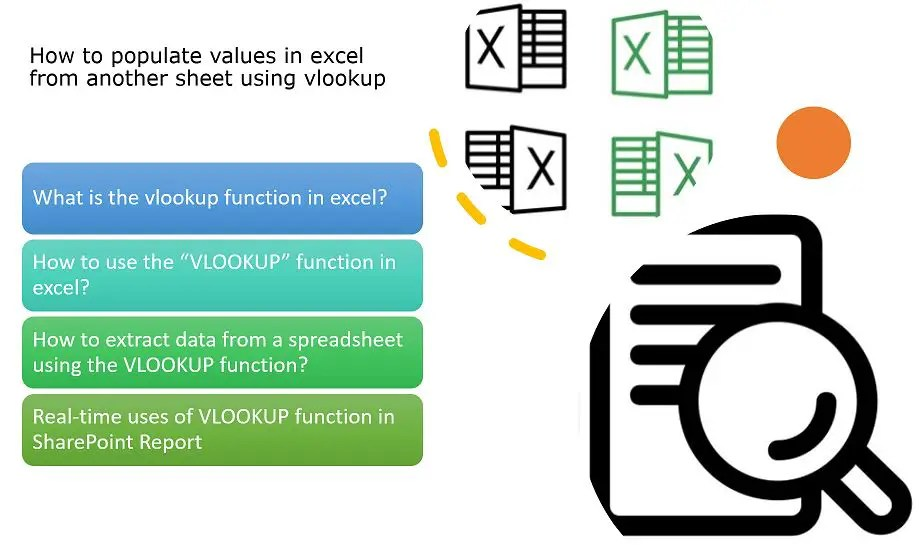# VLOOKUP in Excel: How to populate values in excel from another sheet using vlookupThe VLOOKUP in Excel is one of the much-used functions in excel. Sometimes, while we work in a big excel file that has multiple sheets, then we need to auto-populate data from one sheet to another sheet automatically, recently I was working on a SharePoint Online list inventory report, there I have seen this need. Initially, I started working to fix those mapping or auto-populating manually, then I thought of making this as automation using the VLOOKUP function of excel. This article will show you how to auto-populate values in excel from another sheet using the VLOOKUP function of excel.

## Key-Highlights: VLOOKUP in Excel

• What is the vlookup function in excel?
• How to use the “VLOOKUP” function in excel?
• Real-time uses of VLOOKUP function in SharePoint Report

## What is the vlookup function in excel (VLOOKUP in Excel)?

The VLOOKUP function is one of the most popular functions in Excel, VLOOKUP stands for ‘Vertical Lookup’. It is a function that makes excel search for a certain value in a column (the so-called ‘table array’), in order to return a value from a different column in the same row from the same sheet or different sheet.

In its simplest form, the VLOOKUP function says:

```=VLOOKUP(What you want to look up, where you want to look for it,
the column number in the range containing the value to return,
return an Approximate or Exact match – indicated as 1/TRUE, or 0/FALSE).```

Let’s get started with this implementation.

For this POC (proof of concept) I have used the sample excel with the employee table excel where I have sheets “Employee Details” and “Employee Salary”.

The “Employee Details” sheet has the below two columns:

1. Emp ID
2. Emp NameGet value from one excel sheet to another sheet automatically – Employee Details TableBy the way, the “Employee Salary” sheet structure is as below:

1. Emp ID
2. Get Emp Name from Sheet 1 (Employee Details)
3. Salary
4. Department

In this demo, we will auto-populate the “Emp Name” column values to the “Employee Salary” sheet where intentionally the “Emp Name” column values have been kept empty.

Now using the excel “VLOOKUP” function we will populate the employee name values from the “Employee Details” sheet, below is the formula to get it done.

`=VLOOKUP(A2,'Emloyee Details'!A1:B9,2,TRUE)`

Extract value from one excel sheet to another sheet automatically using the vlookup function

## How to use the “VLOOKUP” function in excel (VLOOKUP in Excel)?

Place the cursor where you want to display the dynamic data, here I have selected the column “B” (Get Emp Name from Sheet 1 (Employee Details)), then follow the below syntax:

```=VLOOKUP(value, table, col_index) //This is to check the approximate match (default)
=VLOOKUP(value, table, col_index, TRUE) //This is to check the approximate match
=VLOOKUP(value, table, col_index, FALSE) //This is to check the exact match```

Explanation about the VLOOKUP with Example:

```=VLOOKUP(select the ID column in from the current sheet,
Select the table - For this go to the previous sheet from where you want to display the data,
Select the column number from the previous sheet which you want to get,
Boolean (True or False for matching result)```

## Real-time uses of VLOOKUP function in SharePoint Report (VLOOKUP in Excel)

When you are working with SharePoint site, list/library, workflow, etc excel report – and wanted to have all these reports in single excel with the various sheets, there we can use the VLOOKUP function.

## Conclusion: VLOOKUP in Excel

Thus, in this article, we have learned about how to use the VLOOKUP function in excel and using the VLOOKUP function how we can automatically get data from another sheet, we can summarize as below:

• What is the vlookup function in excel?
• How to use the “VLOOKUP” function in excel?
• How to extract data from a spreadsheet using the VLOOKUP function?
• Real-time uses of VLOOKUP function in SharePoint Report